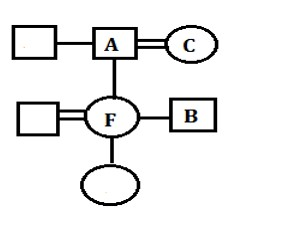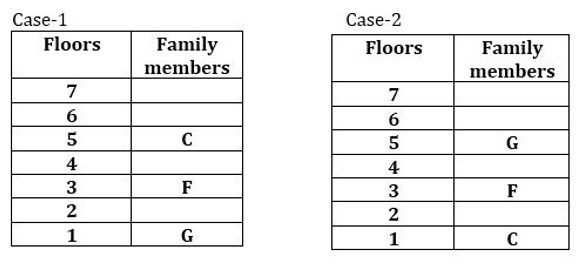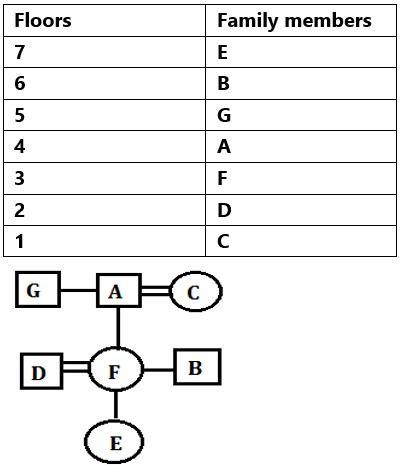# SBI PO Mains Reasoning Ability Quiz – 6

## SBI PO Mains Reasoning Ability Quiz

Reasoning Ability plays a very crucial role in every competitive examination. With consistent practice, candidates can ace this section in examination. In this article, we bring to you SBI PO Mains Reasoning Ability Quiz to boost your preparation. This SBI PO Mains Reasoning Ability Quiz contains various types of questions ranging from easy to difficult level. This SBI PO Mains Reasoning Ability Quiz is absolutely FREE. Candidates will be provided with a detailed explanation of each question in this SBI PO Mains Reasoning Ability Quiz. Question given in this SBI PO Mains Reasoning Ability Quiz are based on the latest or updated exam pattern. In order to be able to answer questions quickly and efficiently in upcoming exams, aspirants must practice this SBI PO Mains Reasoning Ability Quiz.

Direction (1-2): Read the information carefully and answer the questions given below.

Six people viz. A, B, C, D, E, and F live on seven different floors of a building (such as ground floor numbered as 1 and top is numbered as 7 and there is a vacant floor). E lives on an odd numbered floor. F lives just above A, who doesn’t live on an odd numbered floor. C lives on even numbered floor above E’s floor. D lives on an odd numbered floor below E’s floor. As many persons live between B and C as live between E and D. F doesn’t live on 5th floor.

1. Which of the following floor is vacant floor?

(a) second

(b) third

(c) fourth

(d) fifth

(e) None of these

Ans. d

Exp.

(i) E lives on an odd numbered floor. C lives on even numbered floor above E’s floor. D lives on an odd numbered floor below E’s floor, from these condition E can live either on 3rd or 5th floor, So 2 possible case will be there as shown below. F lives just above A, who doesn’t live on an odd numbered floor. F doesn’t live on 5th floor, so in case 1, D lives on 1st floor and F lives on 3rd floor while in case 2 C lives on 4th floor and F lives on 7th floor.(ii) As many persons live between B and C as live between E and D from this condition case 1 will be eliminated. In case 2, B will live on 2nd floor and we will get final answer.1. Who among the following lives on third floor?

(a) C

(b) D

(c) E

(d) F

(e) None of these

Ans. c

Exp.

(i) E lives on an odd numbered floor. C lives on even numbered floor above E’s floor. D lives on an odd numbered floor below E’s floor, from these condition E can live either on 3rd or 5th floor, So 2 possible case will be there as shown below. F lives just above A, who doesn’t live on an odd numbered floor. F doesn’t live on 5th floor, so in case 1, D lives on 1st floor and F lives on 3rd floor while in case 2 C lives on 4th floor and F lives on 7th floor.(ii) As many persons live between B and C as live between E and D from this condition case 1 will be eliminated. In case 2, B will live on 2nd floor and we will get final answer.Directions (3-5): Study the following information and answer the questions given below:

There are four triangles given in question on which we apply some operation individually on each triangle and then convert it into solution. We have to answer the questions from the triangle which comes after the operation applied on the given triangle.

For example-1. For triangle 1- if the addition of place value (alphabetical value) of both the letters is an odd number then change each letter to its second succeeding letter according to alphabetical series.
2. For triangle 2- if the number is perfect square then replace the number with the square of addition of both the digits of that number.
3. For triangle 3- if the addition of place value (alphabetical value) of both the letters is an even number then change each letter to its second preceding letter according to alphabetical series.
4. For triangle 4- if the number is not a perfect square then replace the number with the square of difference of both the digits of that number

Note- If the above conditions are not applied then we write the digit and letter as it is in solution part.

Based on the above example solve the given question.1. How many meaningful words are formed by all the letters combining from triangle 1 and triangle 3 after applying the operation?

(a) None

(b) Two

(c)  Three

(d) One

(e) Four

Ans. a

Exp.

The following steps are followed in the given question-

1- The addition of place value (alphabetical value) of both the letters d and k is an odd number (d=4, k=11), so d and k are changed to f and m respectively.

2- 81 is perfect square so 81 is replaced by the square of addition of both the digits 1+8= 92 =81, hence 81 is changed to 81.

3- The addition of place value (alphabetical value) of both the letters q and e is an even number (q=17, e=5), hence q and e are changed to o and c respectively.

4- 40 is not a perfect square so 40 is replaced by the square of difference of both the digits 4-0=42=16, hence 40 is changed to 16.

The final solution is given below-1. What is the multiplication of numbers which are obtained after applying the operation in triangle 2 and triangle 4?

(a) 1269

(b) 1496

(c) 1396

(d) 1296

(e) None of these

Ans. d

Exp.

The following steps are followed in the given question-

1- The addition of place value (alphabetical value) of both the letters d and k is an odd number (d=4, k=11), so d and k are changed to f and m respectively.

2- 81 is perfect square so 81 is replaced by the square of addition of both the digits 1+8= 92 =81, hence 81 is changed to 81.

3- The addition of place value (alphabetical value) of both the letters q and e is an even number (q=17, e=5), hence q and e are changed to o and c respectively.

4- 40 is not a perfect square so 40 is replaced by the square of difference of both the digits 4-0=42=16, hence 40 is changed to 16.

The final solution is given below-1. Which of the following letters are obtained after applying the operation in triangle 3?

(a) d, k

(b) c, o

(c) o, c

(d) q, f

(e) None of these

Ans. c

Exp.

The following steps are followed in the given question-

1- The addition of place value (alphabetical value) of both the letters d and k is an odd number (d=4, k=11), so d and k are changed to f and m respectively.

2- 81 is perfect square so 81 is replaced by the square of addition of both the digits 1+8= 92 =81, hence 81 is changed to 81.

3- The addition of place value (alphabetical value) of both the letters q and e is an even number (q=17, e=5), hence q and e are changed to o and c respectively.

4- 40 is not a perfect square so 40 is replaced by the square of difference of both the digits 4-0=42=16, hence 40 is changed to 16.

The final solution is given below-Directions (6-10): Study the following information and answer the given questions:

Seven family members A, B, C, D, E, F, and G live on 7 different floors of a building, lowermost floor is numbered as 1 and topmost floor is numbered as 7. There are only 3 female members and 3 generation in a family.  There are three members live between A’s son and A’s son-in-law. F’s daughter lives one of the floors above F’s floor. G lives on an odd numbered floor but not on topmost floor. C is mother of B, who is brother of F, who lives on odd numbered floor. A who is father of F lives above C. The one who lives on topmost floor is not a male member. There are only three floors between G’s floor and C’s floor. A has a sibling. Only two persons live between F’s floor and B’s floor. E lives on an odd numbered floor.

1. Who among the following is daughter of C?

(a) D

(b) E

(c) G

(d) F

(e)None of these

Ans. d

Exp.

(i) To solve such kind of puzzle first we have to try to make family tree by using the given conditions. There are three members live between A’s son and A’s son-in-law. F’s daughter lives one of the floors above F’s floor, it means F has a daughter. A who is father of F. C is mother of B, who is brother of F, it means A is married to C. A has a sibling, hence F will daughter of A, this is the only possibility is left for F. A has a sibling, so A can have brother or sister, but it is given that only 3 female members are there so A will have a brother.(ii) In a family tree positions of D, E, and G are not fixed, to fix their positions, try to solve the puzzle with floors arrangement by using remaining conditions.

(iii)  G lives on an odd numbered floor but not on topmost floor. There are only three floors between G’s floor and C’s floor. A who is father of F lives above C. F’s daughter lives one of the floors above F’s floor, so F can’t live on 7th floor and F lives on odd numbered floor, so F’s position will be fixed that is on 3rd floor, by using these conditions only 2 possibilities will be there-(iv) Only two persons live between F’s floor and B’s floor, so B lives on 6th floor. B is son of A and it is given that three members live between A’s son (B) and A’s son-in-law, who will live on 2nd floor. E lives on odd numbered floor, hence E lives on 7th floor only this is possible place is left for E. A will live on 4th floor and D will live on 2nd floor, hence D is son in law of A.(v) The one who lives on topmost floor is not a male member, hence E is female and is a daughter of F and G is brother of A. A who is father of F lives above C, from this condition case 2 will be eliminated. Finally, we get the final solution.1. Who amongst the following lives on floor number 3?

(a) B’s daughter

(b) D

(c) E

(d) D’s wife

(e) None of these

Ans. d
1. C lives on which of the following floor?

(a) 4th floor

(b) 6th floor

(c) 5th floor

(d) None of these

(e) 1st floor

Ans. e
1. Who among the following is uncle of F?

(a) E

(b) C

(c)  G

(d) Both (a) and (b)

(e) None of these

Ans. c
1. How many people live between the floors on which D and B live?

(a) None

(b) Two

(c) One

(d) More than three

(e) Three